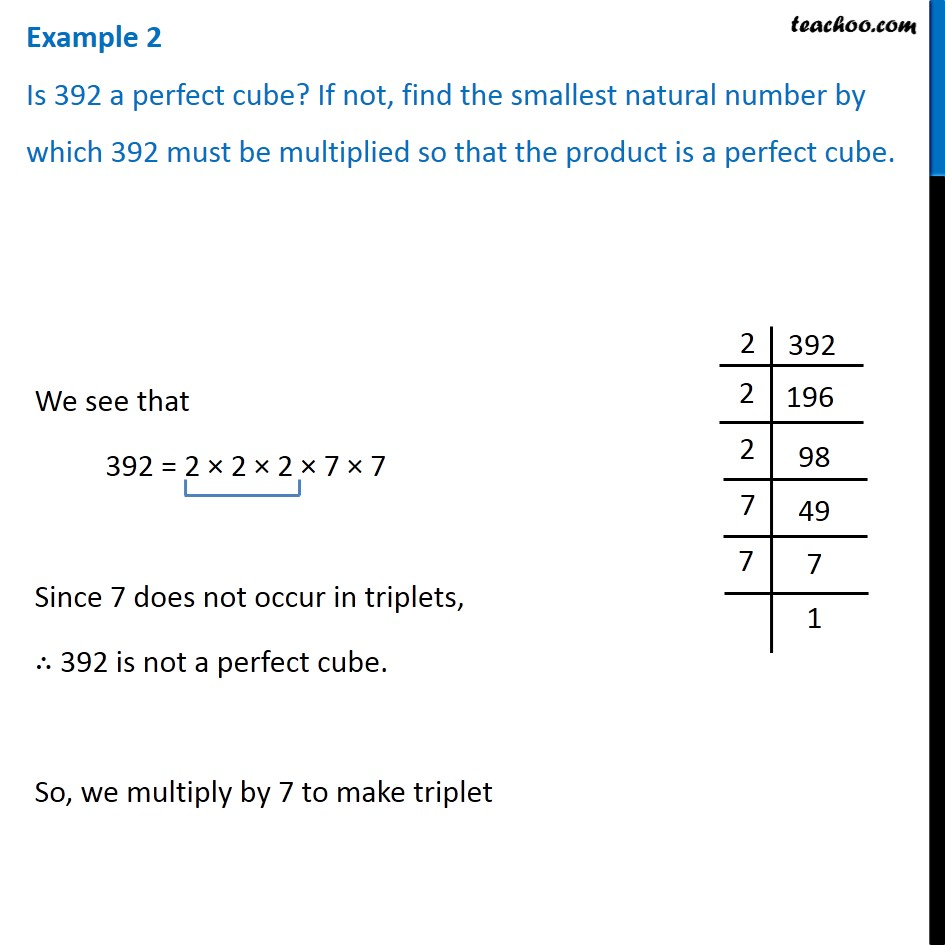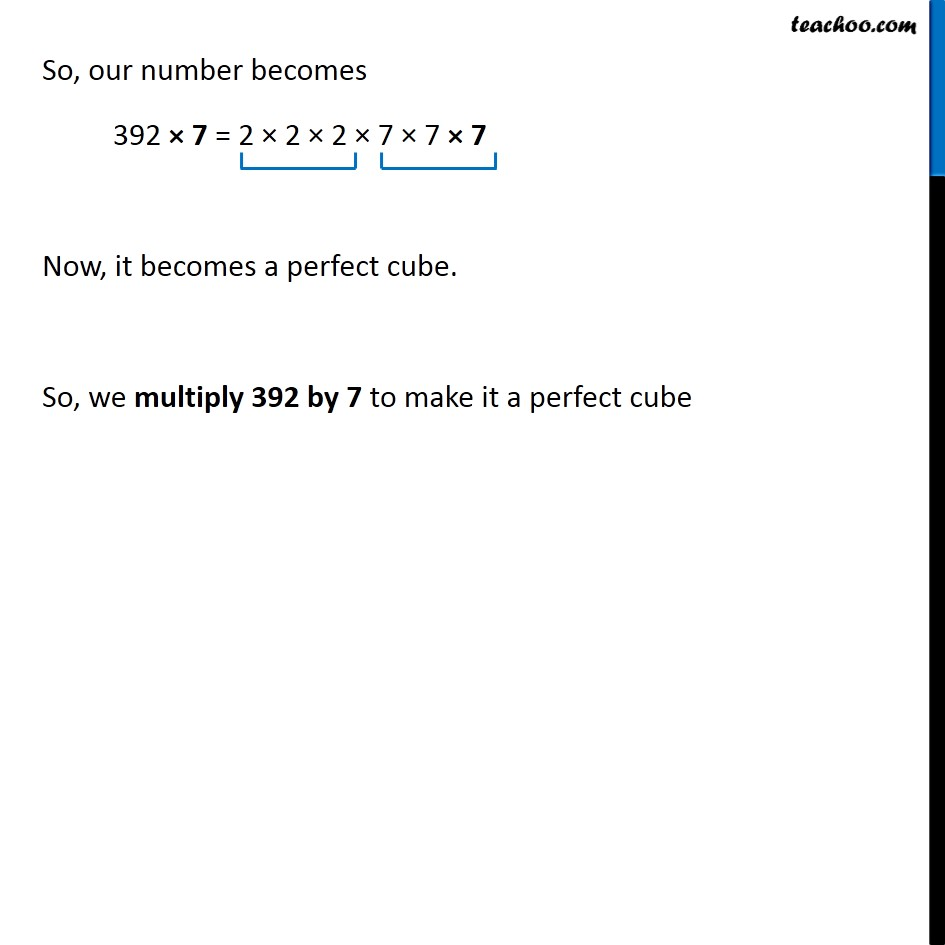Subscribe to our Youtube Channel - https://you.tube/teachoo

1. Chapter 7 Class 8 Cubes and Cube Roots
2. Concept wise
3. Smallest number multiplied to get a perfect cube

Transcript

Example 2 Is 392 a perfect cube? If not, find the smallest natural number by which 392 must be multiplied so that the product is a perfect cube. We see that 392 = 2 × 2 × 2 × 7 × 7 Since 7 does not occur in triplets, ∴ 392 is not a perfect cube. So, we multiply by 7 to make triplet So, our number becomes 392 × 7 = 2 × 2 × 2 × 7 × 7 × 7 Now, it becomes a perfect cube. So, we multiply 392 by 7 to make it a perfect cube

Smallest number multiplied to get a perfect cube